# MP Board Class 6th Maths Solutions Chapter 3 Playing With Numbers Ex 3.7

## MP Board Class 6th Maths Solutions Chapter 3 Playing With Numbers Ex 3.7

Question 1.
Renu purchases two bags of fertiliser of weights 75 kg and 69 kg. Find the maximum value of weight which can measure the weight of the fertiliser exact number of times.
Solution:
For finding maximum weight, we have to find HCF of 75 and 69.
The prime factorisation of 75 and 69 are;
75 = 3 × 5 × 5
69 = 3 × 23
So, HCF of 75 and 69 = 3
Therefore, the required weight is 3 kg.Question 2.
Three boys step off together from the same spot. Their steps measure 63 cm, 70 cm and 77 cm respectively. What is the minimum distance each should cover so that all can cover the distance in complete steps?
Solution:
For finding minimum distance, we have to find LCM of 63, 70, 77.L.C.M. of 63, 70 and 77 = 2 × 3 × 3 × 5 × 7 × 11
= 6930
Therefore, the minimum distance three boys should cover is 6930 cm.Question 3.
The length, breadth and height of a room are 825 cm, 675 cm and 450 cm respectively. Find the longest tape which can measure the three dimensions of the room exactly.
Solution:
The measurement of the longest tape = HCF of 825 cm, 675 cm and 450 cm are;
825 = 3 × 5 × 5 × 11
675 = 3 × 3 × 3 × 5 × 5
450 = 2 × 3 × 3 × 5 × 5
Now, HCF of 825, 675 and 450 = 3 × 5 × 5 = 75
Therefore, the measurement of longest tape is 75 cmQuestion 4.
Determine the smallest 3-digit number which is exactly divisible by 6, 8 and 12.
Solution:
The smallest 3-digit number = 100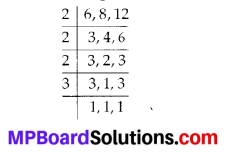L.C.M of 6, 8 and 12 = 2 × 2 × 2 × 3 = 24
To find the number, we have to divide 100 by 24.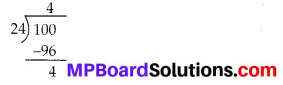Therefore, the required number = 100 + (24 – 4) = 120.

Question 5.
Determine the greatest 3-digit number exactly divisible by 8,10 and 12.
Solution:
The greatest three digit number = 999.L.C.M of 8, 10 and 12 = 2 × 2 × 2 × 3 × 5 = 120
Now, to find the number, we have to divide 999 by 120.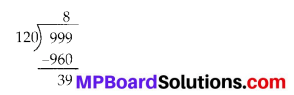Therefore the required number = 999 – 39 = 960Question 6.
The traffic lights at three different road crossings change after every 48 seconds, 72 seconds and 108 seconds respectively. If they change simultaneously at 7 a.m., at what time will they change simultaneously again?
Solution:LCM of 48, 72 and 108 = 2 × 2 × 2 × 2 × 3 × 3 × 3 = 432
After 432 seconds, the traffic lights change simultaneously.
432 seconds = 7 minutes 12 seconds
Therefore, the required time = 7 a.m. + 7 minutes 12 seconds i.e., 7 minutes 12 seconds past 7 a.m.

Question 7.
Three tankers contain 403 litres, 434 litres and 465 litres of diesel respectively. Find the maximum capacity of a container that can measure the diesel of the three containers exact number of times.
Solution:
The maximum capacity of a container = HCF (403, 434, 465)
The prime factorisation of 403, 434 and 465 are;
403 = 13 × 31
434 = 2 × 7 × 31
465 = 3 × 5 × 31
HCF of 403, 434, 465 = 31
Therefore, a container of capacity 31 litres is required to measure the diesel of the three containers.Question 8.
Find the least number which when divided by 6,15 and 18 leave remainder 5 in each case.
Solution:LCM of 6,15 and 18 = 2 × 3 × 3 × 5 = 90
Therefore, the required number = 90 + 5 = 95

Question 9.
Find the smallest 4-digit number which is divisible by 18, 24 and 32.
Solution:
The smallest four digit number = 1000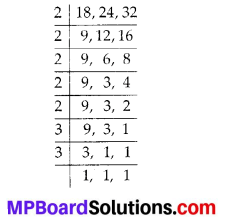LCM of 18, 24 and 32 = 2 × 2 × 2 × 2 × 2 × 3 × 3 = 288
Now,Therefore, the required number is 1000 + (288 – 136) = 1152.Question 10.
Find the LCM of the following numbers :
(a) 9 and 4
(b) 12 and 5
(c) 6 and 5
(d) 15 and 4
Observe a common property in the obtained LCMs. Is LCM the product of two numbers in each case?
Solution:
(a) We have,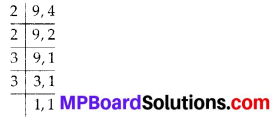LCM of 9 and 4 = 2 × 2 × 3 × 3 = 36

(b) We have,LCM of 12 and 5 = 2 × 2 × 3 × 5 = 60

(c) We have,LCM of 6 and 5 = 2 × 3 × 5 = 30

(d) We have,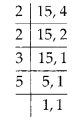LCM of 15 and 4 = 2 × 2 × 3 × 5 = 60
Yes, the LCM is equal to the product of two numbers in each case and all LCMs are also the multiple of 3.

Question 11.
Find the LCM of the following numbers in
(a) 5, 20
(b) 6, 18
(c) 12, 48
(d) 9, 45
What do you observe in the results obtained?
Solution:
(a) We have,LCM of 5 and 20 = 2 × 2 × 5 = 20

(b) We haveLCM of 6 and 18 = 2 × 3 × 3 = 18

(c) We haveLCM of 12 and 48 = 2 × 2 × 2 × 2 × 3 = 48

(d) We have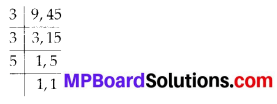LCM of 9 and 45 = 3 × 3 × 5 = 45
From above, we observe that the LCM of the given numbers in each case is the larger of the two numbers.